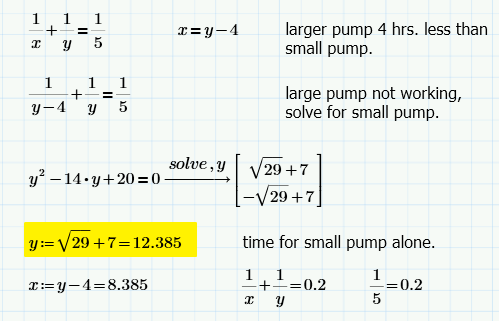# Emptying a Tank

4 posts / 0 new
ramelcoletana
Emptying a Tank

Two pumps of different sizes, working
together, can empty a fuel tank in 5 hours.The larger pump
can empty this tank in 4 hours less than the smaller one. If the
larger pump is out of order, how long will it take the smaller
one to do the job alone?

Tags:
JoeJhun VertIs this a MathCAD? The symbol := is familiar.

Engr JaydeeYes sir, I think this is MathCAD Prime.

• Mathematics inside the configured delimiters is rendered by MathJax. The default math delimiters are $$...$$ and $...$ for displayed mathematics, and $...$ and $...$ for in-line mathematics.